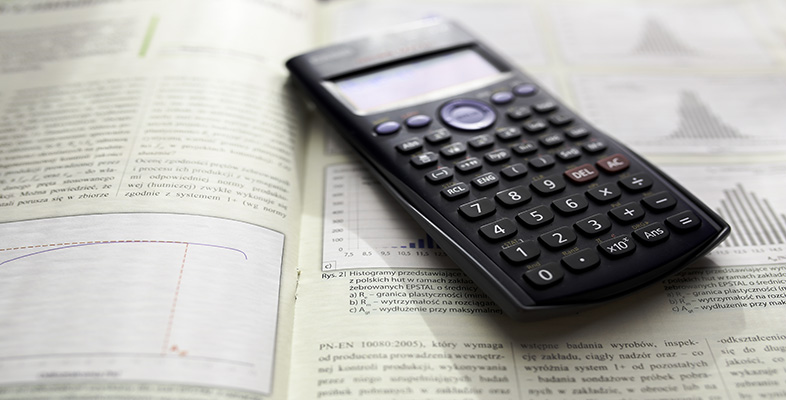Using a scientific calculator

This free course is available to start right now. Review the full course description and key learning outcomes and create an account and enrol if you want a free statement of participation.

Free course

3 Using your calculator for fractions

When your calculator is in Math mode, as recommended, fractions are entered using the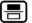button in the left-hand column of the function key area of the calculator keypad. This displays a fraction ‘template’ on the display – as shown in Figure 8 below – that contains boxes that need to be ‘filled in’. When the button is first pressed, the cursor is located in the top box ready for you to enter the numerator. To move to the bottom box to enter the denominator, use the cursor down key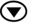. If there are further parts of a calculation to be entered when the template has been completed, the right cursor key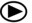can be used to move out of the denominator in preparation for the input of the rest of the calculation.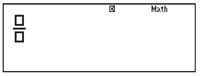Figure 8 A fraction template

Remember, your calculator is in Math mode if the word Math is shown at the top of the calculator display. If your calculator is not in Math mode, repeat the steps of Activity 1.

Mixed numbers such as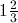can be entered similarly using the mixed number template obtained using the key sequence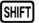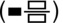. This template provides three boxes to fill, one for the whole number part, and one each for the numerator and denominator of the fractional part.

Any fractional answers to calculations will automatically be displayed in lowest terms.

Activity 7 Fractions

1. express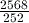in its simplest form
2. calculate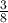of 190.

1.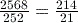2.of 190 can be written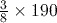, which is equal to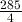or 71.25.

The decimal answer can be obtained by using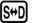.

Remember to use the cursor right keyto move the cursor out of the denominator of the fraction before entering the multiplication sign. If you obtained the answer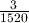, you calculated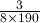by mistake.

You may have noticed that the results of both these exercises were displayed on the calculator as top-heavy fractions. This is the default behaviour of the calculator in Math mode. You can toggle between a top-heavy fraction and its mixed number equivalent using the key sequence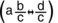.

The default behaviour of the calculator can be changed as follows:

• To set the calculator to use mixed numbers by default, use the key sequence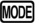(SETUP)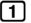(ab/c).
• To set the calculator to use improper or top-heavy fractions by default, use the key sequence(SETUP)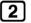(d/c).

Here, thekey is used to access part of the on-screen menu that is not initially visible.

Activity 8 Mixed numbers

1. expressas a mixed number in its simplest form
2. express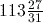as a top-heavy fraction.

1.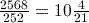Remember to useto toggle between the top-heavy fraction and mixed number answers.

2.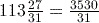Remember to use the template obtained usingand to use the cursor arrow keys to move between the boxes when inputting the mixed number.

MU123_1